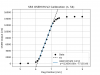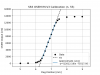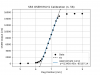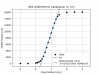Reports 1-1 of 1
VIS (SR3)
enzo.tapia - 14:18 Thursday 26 April 2018 (4846) Print this report
SR3 IM OSEM calibration

With Kuzu-kun.

We inserted a filter in FM1 called mV/cnt with a gain of 20/2^16 [V/cnt]=20000/2^16 [V/cnt] = 0.305175[mV/cnt]. This represents the electrical gain of the ADC. Then we can divide the calibration factor by FM1. This gives values of a more convenient magnitude for typing in the gain box.

---------OSEM No. 53-------------- (IM-V1)

Max. output: 14218[cnt] ----> Offset: -7109.0

Calibration factor: 1/(12659.7 [cnt/mm]) = 0.078991 [µm/cnt] ---->  0.078991 [µm/cnt]/FM1  = 0.259 [µm/mV]

Linear range: 1600-12500 [cnt]

---------OSEM No. 54-------------- (IM-V2)

Max. output: 14850 [cnt] ----> Offset: -7425.0

Calibration factor: 1/(12656.0 [cnt/mm]) = 0.079014 [µm/cnt] ---->  0.079014 [µm/cnt]/FM1  = 0.259 [µm/mV]

Linear range: 1480-12600 [cnt]

---------OSEM No. 55-------------- (IM-V3)

Max. output: 13870 [cnt] ----> Offset: -6935.0

Calibration factor: 1/(12012.18 [cnt/mm]) = 0.083248 [µm/cnt] ---->  0.083248 [µm/cnt]/FM1 = 0.273 [µm/mV]

Linear range: 1500-12000 [cnt]

---------OSEM No. 56-------------- (IM-H1)

Max. output: 15741 [cnt] ----> Offset: -7870.5

Calibration factor: 1/(13450.45 [cnt/mm]) = 0.074347 [µm/cnt] ---->  0.074347 [µm/cnt]/FM1 = 0.244 [µm/mV]

Linear range: 1390-14200 [cnt]

---------OSEM No. 57-------------- (IM-H2)

Max. output: 16632 [cnt] ----> Offset: -8316.0

Calibration factor: 1/(14133.09 [cnt/mm]) = 0.070756 [µm/cnt] ---->  0.070756 [µm/cnt]/FM1 = 0.232 [µm/mV]

Linear range: 1370-14700 [cnt]

---------OSEM No. 58-------------- (IM-H3)

Max. output: 12603 [cnt] ----> Offset: -6301.5

Calibration factor: 1/(11272.55 [cnt/mm]) = 0.088711 [µm/cnt] ---->  0.070756 [µm/cnt]/FM1 = 0.291 [µm/mV]

Linear range: 1450-11100 [cnt]

After inserting this values into the digital system I snapshotted the settings.

Note: Kozu-kun noticed that at open light the Osem nr. 53 and nr. 54 exhibit a long settle down time.... about 8 minutes.

Images attached to this report×

×